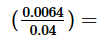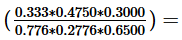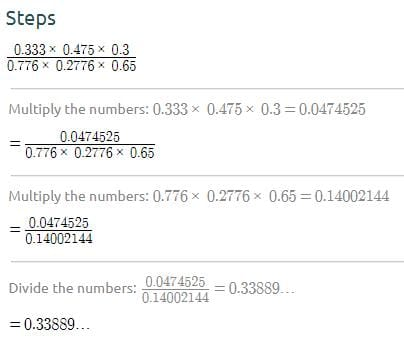# Test: Digits And Rounding Off

## 10 Questions MCQ Test Quantitative Reasoning for GMAT | Test: Digits And Rounding Off

Description
Attempt Test: Digits And Rounding Off | 10 questions in 20 minutes | Mock test for GMAT preparation | Free important questions MCQ to study Quantitative Reasoning for GMAT for GMAT Exam | Download free PDF with solutions
QUESTION: 1

### In the decimal, 1.2d9, d represents a digit from 0 to 9. If the value of the decimal rounded to the nearsest tenth is less than 1.3, what are the possible value of d?

Solution:

If the final result is less than 1.3, it means that the result is 1.2. If the result is 1.2, the previous digit has been kept the same. According to the rounding off rules, to keep the previous digit the same, the next digit should be less than 5. can be 0, 1, 2, 3 or 4. The answer is C

QUESTION: 2

###Solution:

0.0064/0.04 = (64/10000)/(4/100)
= (64/10000) * (100/4)
= 16/100
= 0.16

QUESTION: 3

### If x is an integer and 2.134 × 10^x is less than 210,000, what is the greatest possible value for x?

Solution:

for 2.134 × 10x is less than 210,000 to remain true, the greatest number is 21,340, which makes x=4

QUESTION: 4

By evaluating (83 x 2.06)⁄5.48, answer correct to three significant figures is

Solution:
QUESTION: 5

What is 234,567 ÷ 103, rounded to the nearest whole number?

Solution:

234,567 ÷ 103 = 234.567.

According to the rounding off rules, to round off to the nearest integer, look for the first digit after decimal point. The first digit after the decimal is 5 (which is more than equal to 5) so the answer would be 235. The answer is B.

QUESTION: 6

In the number 1.3ab8, a and b respresent single positive digit. If x = 1.3ab8, what is the value of 10 - x?

1) If x is rounded to the nearest hundredth, then 10 - x = 8.66

2) If x is rounded to the nearest thousandth, then 10 - x = 8.664

Solution:

To find the value of 10 - x, find the value of a and b.

Considering statement (1) alone:
10 - x = 8.66

⇒ x = 10 - 8.66

⇒  x = 1.34
Comparing this value with 1.3ab8, the only information we get is that a can be either 3 or 4. Insufficient

Considering statement (2) alone:
10 - x = 8.664

⇒  x = 10 - 8.664

⇒ x = 1.336
Comparing this value with 1.3ab8, it can be infered that a = 3 and b = 5 (using the rules of rounding off).  Sufficient

QUESTION: 7

The correct value of 0.006472867 up to three significant figures is

Solution:
QUESTION: 8

Which of the following is more than 3/4?

Solution:

Cross multiply and check.

or

Calculate and check

QUESTION: 9

What is the decimal equivalent of (2/5)3?

Solution:

Calculate

QUESTION: 10Solution:Use Code STAYHOME200 and get INR 200 additional OFF Use Coupon Code